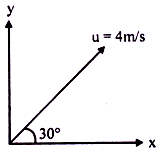# The answer to each of the questions is a single digit integer ranging from 0 to 9.     A very broad elevator is going up vertically with a constant acceleration of 2 m/s2. At the instant when its velocity is 4 m/s a ball is projected from the floor of the lift with a speed of 4 m/s relative to the floor at an elevation of 30o. The time taken by the ball to return the floor issecond. Find x. (g = 10 m/s2)9 years ago

3

Components of velocity of ball relative to lift are

ux = 4 cos 30o = 2√3 m/s

and    uy = 4 sin 30o = 2 m/s

T = (2u_y)/12=u_y/6=2/6=1/3 s

x = 35 years ago
Total acceleration=g+ 2m/s=12 m/sTherefore time of flight=(2usinΦ)/g=(2*4*1/2)/12 =1/3 s. Given [u=4m/s ; sin30=1/2]Hope this helps.... If you have any problem regarding this... Post it here.....
5 years ago
A very broad elevator is going up vertically with a constant acceleration of 2 m/s2. At the instant when its velocity is 4 m/s a ball is projected from the floor of the lift with a speed of 4 m/s relative to the floor at an elevation of 30o. The time taken by the ball to return the floor issecond. Find x. (g = 10 m/s2)
5 years ago
otal acceleration=g+ 2m/s=12 m/sTherefore time of flight=(2usinΦ)/g=(2*4*1/2)/12 =1/3 s. Given [u=4m/s ; sin30=1/2]Hope this helps.... If you have any problem regarding this... Post it here.....nbk